# K. G Sreekumar

•### Equation of straight line with two given points

Activity

K. G Sreekumar

•Activity

K. G Sreekumar

•### Concavity of graphs of functions - Concave up and down

Activity

K. G Sreekumar

•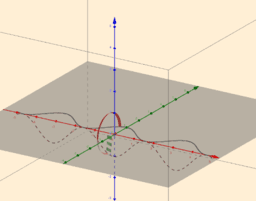### Volume of Solids by revolution (nct1)

Activity

K. G Sreekumar

•### Relation between function and its derivative(Nct2)

Activity

K. G Sreekumar

•### Comparison between different Riemann Integrals

Activity

K. G Sreekumar

•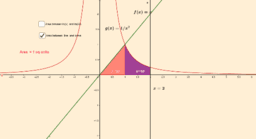### Area between lines and curves

Activity

K. G Sreekumar

•### Area between parabola and a curve

Activity

K. G Sreekumar

•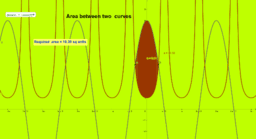### Area between two curves

Activity

K. G Sreekumar

•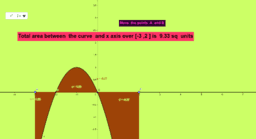### Area under a curve

Activity

K. G Sreekumar

•### Derivative of implicitly defined functions

Activity

K. G Sreekumar

•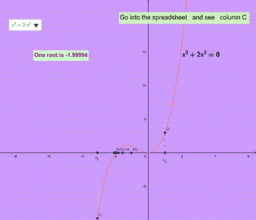### Bisection method to find a real root of an equation

Activity

K. G Sreekumar

•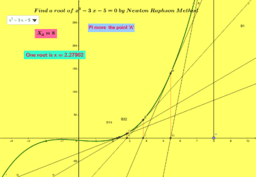### Newton Raphson method

Activity

K. G Sreekumar

•### Orthogonal trajectories self

Activity

K. G Sreekumar

•### Orthogonal trajectories 1

Activity

K. G Sreekumar

•### Solution of ODE and integration - Examples

Activity

K. G Sreekumar

•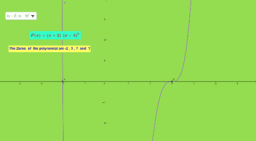### Zeros of Polynomials- Example

Activity

K. G Sreekumar

•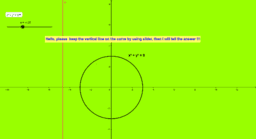### Vertical line test

Activity

K. G Sreekumar

•### Determine Which of the following equations define functions

Activity

K. G Sreekumar

•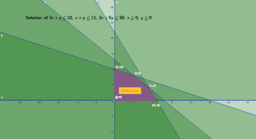### Solution of Linear inequalities in two variables

Activity

K. G Sreekumar

•Activity

K. G Sreekumar

•### Linear functions -Examples

Activity

K. G Sreekumar

•### Inverse of functions -Examples

Activity

K. G Sreekumar

•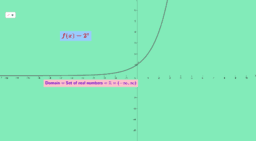### Exponential and logarithmic functions

Activity

K. G Sreekumar